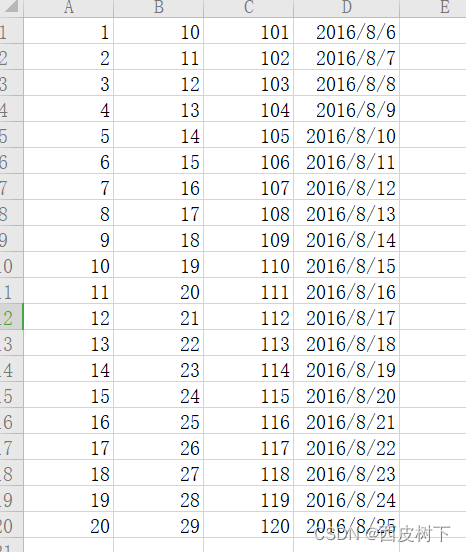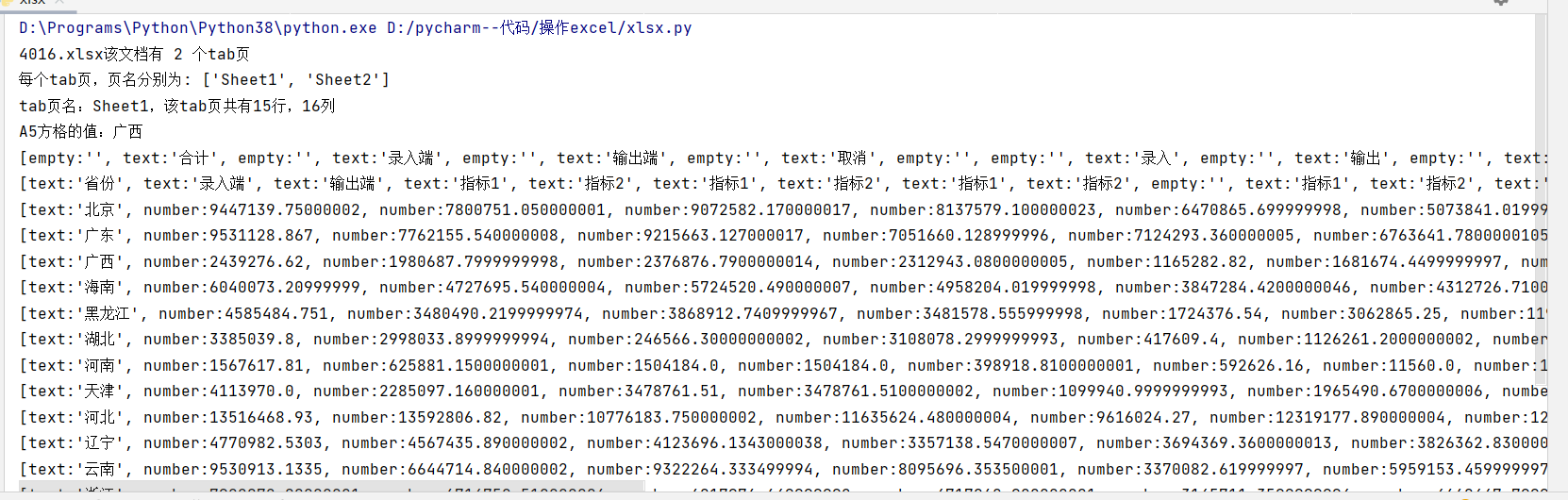• python读取excel文件数据
千次阅读
2021-01-27 16:40:49

Python读取excel文件数据并画柱状图

import xlrd
import os
import numpy as np
import matplotlib.pyplot as plt
import math

data = {}

workBook = xlrd.open_workbook(file_path)
allSheetNames = workBook.sheet_names()

for sheet_name in allSheetNames:
temp_data = {}
sheet_content = workBook.sheet_by_name(sheet_name)
rows = sheet_content.nrows
for row in range(rows):
row_data = sheet_content.row_values(row)
if row == 0:
temp_data['type'] = row_data[2:]
else:
temp_data[row_data] = row_data[2:]

data[sheet_name] = temp_data
return data

def show_figure(data, type):

sheet_content = data[type]
num_x = np.arange(len(sheet_content['type']))
fig, ax = plt.subplots()
width = 0.1
num_width = 0
for k in sheet_content:
if k == 'type':
continue
y = sheet_content[k]
for i in range(len(y)):
if y[i] == 'null':
y[i] = 0
y = [i*100 for i in y]
ax.bar(num_x + num_width * width, y, width, alpha=1, label=k)
num_width += 1
ax.set_xticks(num_x + (num_width - 1) * width / 2)
ax.set_xticklabels(sheet_content['type'], fontsize=15)
ax.set_ylabel('MMrTRE [%]', fontsize=15)
#ax.set_title(type, fontsize=25)
ax.legend(fontsize=12, ncol=3)
ax.set_yscale('log')

plt.yticks(fontsize=15)
plt.grid()
plt.show()

if __name__ == '__main__':
repoRoot = os.path.dirname(os.path.realpath(__file__))
file_path = os.path.join(repoRoot, 'ANHIR_metric.xlsx')

# show figure
show_figure(data, 'All')
show_figure(data, 'Evaluation')

更多相关内容
• 本文使用xlrd读取excel文件（xls,sxls格式)，使用xlwt向excel写入数据 一、xlrd和xlwt的安装 安装很简单，windos+r调出运行窗口，输入cmd，进入命令行窗口，输入以下命令。 安装xlrd: pip install xlrd 安装xlwt: ...
• 主要介绍了python 读取excel文件生成sql文件实例详解的相关资料,需要的朋友可以参考下
• 目的：将excel文件StudentInfo.xls的学生信息插入到test库中的student表中 一、连接mysql数据库 安装第三方库pymysql：pip install pymysql 调用pymysql.connect()方法连接数据库，代码如下 import pymysql # ...

例子： 将excel文件StudentInfo.xls的学生信息插入到student表中注: 使用的版本：Python3.7，MySQL5.5

一、连接mysql数据库

1. 安装第三方库pymysql：pip install pymysql（Python2中则使用mysqldb）
2. 调用pymysql.connect()方法连接数据库，代码如下
import pymysql

# 打开数据库连接
conn = pymysql.connect(
host='localhost',  # MySQL服务器地址
user='root',  # MySQL服务器端口号
charset='utf8',  # 密码
port=3308,  # 端口
db='test',  # 数据库名称
)

# 使用cursor()方法获取操作游标
c = conn.cursor()
sql = "show databases"
# 使用execute方法执行SQL语句
c.execute(sql)
# 使用 fetchone() 方法获取一条数据
res = c.fetchone()
print(res)
# 关闭数据库连接
conn.close()


3.运行程序，如果控制台没有报错，且能正常执行sql语句，则代表连接数据库成功；1. 连接数据库成功后，先插入一条数据看看效果 👇
# 使用cursor()方法获取操作游标
c = conn.cursor()
sql = "insert into student(Sno,Sname,Ssex,Sage,Sdept) value ('2012151','刚子','男','29','CS')"
# 使用execute方法执行SQL语句
c.execute(sql)
# 插入数据,需执行conn.commit()
conn.commit()
# 关闭数据库连接
conn.close()


注：使用pymysql操作数据库时，增删改与查询是有区别的，在增删改操作时一定要记得conn.commit()，提交当前事务。二、读取excel文件

1. 读取excel文件需要用到xlrd库，安装方法：pip install xlrd
2. 对excel文件中的数据进行读取 👇
import xlrd

FilePath = 'E:/PDBC/StudentInfo.xls'

# 1.打开excel文件
wkb = xlrd.open_workbook(FilePath)
# 2.获取sheet
sheet = wkb.sheet_by_index(0)  # 获取第一个sheet表['学生信息']
# 3.获取总行数
rows_number = sheet.nrows
# 4.遍历sheet表中所有行的数据，并保存至一个空列表cap[]
cap = []
for i in range(rows_number):
x = sheet.row_values(i)  # 获取第i行的值（从0开始算起）
cap.append(x)
print(cap)1. 上面读取到的结果为列表类型，每个小列表代表一个学生的信息。因为student表中有五个字段，分别是：Sno、Sname、Ssex、Sage、Sdept，所以我们要拿到每个学生的这五个属性值 👇
for Stu in cap:
Sno = int(Stu)
Sname = Stu
Ssex = Stu
Sage = Stu
Sdept = Stu
print(Sno, Sname, Ssex, Sage, Sdept)三、批量插入数据库

获取到每个学生的属性值后，就可以逐个插入到数据中了，总代码如下

import pymysql
import xlrd

"""
一、连接mysql数据库
"""
# 打开数据库连接
conn = pymysql.connect(
host='localhost',  # MySQL服务器地址
user='root',  # MySQL服务器端口号
charset='utf8',  # 密码
port=3308,  # 端口
db='test',  # 数据库名称
)

# 使用cursor()方法获取操作游标
c = conn.cursor()

"""
二、读取excel文件
"""
FilePath = 'E:/PDBC/StudentInfo.xls'

# 1.打开excel文件
wkb = xlrd.open_workbook(FilePath)
# 2.获取sheet
sheet = wkb.sheet_by_index(0)  # 获取第一个sheet表['学生信息']
# 3.获取总行数
rows_number = sheet.nrows
# 4.遍历sheet表中所有行的数据，并保存至一个空列表cap[]
cap = []
for i in range(rows_number):
x = sheet.row_values(i)  # 获取第i行的值（从0开始算起）
cap.append(x)
print(cap)  # [['9022478', '郭赛', '男', 34.0, 'CS'], ['9022472', '林伟', '男', 36.0, 'MA'], ···]

"""
三、将读取到的数据批量插入数据库
"""
for Stu in cap:
Sno = int(Stu)
Sname = Stu
Ssex = Stu
Sage = Stu
Sdept = Stu
# 使用f-string格式化字符串，对sql进行赋值
c.execute(f"insert into student(Sno,Sname,Ssex,Sage,Sdept) value ('{Sno}','{Sname}','{Ssex}','{Sage}','{Sdept}')")
conn.commit()
conn.close()
print("插入数据完成！")展开全文mysql
• python入门级读取excel文件数据并且画折线图

1.excel表的数据结构2.代码截图

2.1先读取excel数据创建空list数组存储数据

2.2读取col为每一列数据选取然后自定义选取x轴的数据，这里选择了行数为x

2.3读取一个工作簿中的每一行数据

2.4将list数组，存储读取的初始数据

2.5读取每一行中的每列数据

2.6将读取到的数据添加到values数组中

2.7添加网格，方便观察数据图像

2.8添加x和y坐标轴信息，和图例说明

2.9为了图像显示正常需要解决python中文问题

2.10为图像清晰说明需要添加必要的文字说明

import matplotlib.pyplot as plt
import xlrd
xpix_data = []
x_data = []
y_data = []
z_data = []
wb = xlrd.open_workbook('2.xls')
for s in wb.sheets():
for row in range(s.nrows):
xpix_data.append(row)
values = []
for col in range(s.ncols):
values.append(s.cell(row, col).value)
x_data.append(values)
y_data.append(values)
z_data.append(values)
plt.grid()
plt.plot(xpix_data, x_data,label='x轴数据')
plt.plot(xpix_data, y_data,label='y轴数据')
plt.plot(xpix_data, z_data,label='z轴数据')
plt.rcParams['font.sans-serif']=['SimHei']
plt.rcParams['axes.unicode_minus'] = False
plt.title(u"日常数据波形")
plt.xlabel(u"数据时间先后序列")
plt.ylabel(u"数据波动值")
plt.legend()
plt.show()



3.将上面代码运行结果得出图片。

展开全文开发语言
• 主要为大家详细介绍了python读取各种文件数据方法，具有一定的参考价值，感兴趣的小伙伴们可以参考一下
• python读取excel文件的内容可以方便我们查看信息。 # -*- codeing = utf-8 -*- # @Time : 2022/4/16 21:06 # @Author : ning # @File : xlsx.py # @software : PyCharm # 引入xlrd2去支持读取xlsx相关的文件 ...

python读取excel文件的内容可以方便我们查看信息。

# -*- codeing = utf-8 -*-
# @Time : 2022/4/16 21:06
# @Author : ning
# @File : xlsx.py
# @software : PyCharm
# 引入xlrd2去支持读取xlsx相关的文件
import xlrd2
# 定义文件名
file_name = '4016.xlsx'
# 读取xlsx文件
# 预计输出
# 4016.xlsx该文件有 2 个tab页
sku_file = xlrd2.open_workbook(file_name)
print("{0}该文档有 {1} 个tab页".format(file_name, sku_file.nsheets))
print("每个tab页，页名分别为: {0}".format(sku_file.sheet_names()))

# 读取xlsx文件第1页
# 预计输出
# tab页名：Sheet1，该tab页共有15行，16列
# A5方格的值：广西
current_sheet_index = 0  # 下标0为第一页tab
current_sheet = sku_file.sheet_by_index(current_sheet_index)
print("tab页名：{0}，该tab页共有{1}行，{2}列".format(current_sheet.name, current_sheet.nrows, current_sheet.ncols))
print("A5方格的值：{0}".format(current_sheet.cell_value(rowx=4, colx=0)))

# 打印每页的数据，每一行的数据为一个数组
# 预计输出
# [empty:'', text:'合计', empty:'', text:'录入端', empty:'', text:'输出端', empty:'', text:'取消', empty:'', empty:'', text:'录入', empty:'', text:'输出', empty:'', text:'取消', empty:'']
# [text:'省份', text:'录入端', text:'输出端', text:'指标1', text:'指标2', text:'指标1', text:'指标2', text:'指标1', text:'指标2', empty:'', text:'指标1', text:'指标2', text:'指标1', text:'指标2', text:'录入端', text:'输出端']
# ...以下省略后续打印
for rx in range(current_sheet.nrows):
print(current_sheet.row(rx))

结果如下展开全文list
• 主要介绍了python3 读取Excel表格中的数据的相关资料,需要的朋友可以参考下
• 通过Python程序读取Excel文件中的数据，生成图表，最好将生成图表生成至浏览器页面，后期数据多之后，也能自动缩放，而不会出现显示不全问题。 三、需求实现代码 # 调用本地echarts.min.js 文件 from pyecharts....
• 主要介绍了Python读取excel文件中带公式的值的实现，文中通过示例代码介绍的非常详细，对大家的学习或者工作具有一定的参考学习价值，需要的朋友们下面随着小编来一起学习学习吧
• import openpyxl import datetime import os from os.path import join, getsize now_time = datetime.datetime.now() yesterday = (now_time + datetime....# 本地数据存放地址 base_dir = '/home/hkxpz/ceshu/' ...
• 但是当横轴是日期时，且从excel文件读取数据时，有一点麻烦。 遇到的问题： 1. 首先，excel里的日期显示不正常。 2. 横轴日期的显示不理想，或太密集或太松弛。 解决： 1. 方法一：修改excel数据类型 excel里的日期...
• ## Python读取Excel文件

千次阅读 2022-04-10 15:18:30
• 1.引入库 xlrd import xlrd 2.打开文件 workBook = xlrd.open_workbook(r'文件位置/名字.xlsx') 3.获取sheet名字 ...# 1.1 获取所有sheet的名字(list类型) ...def read_excel(): # 打开文件 workBook
• 本文实例讲述了python实现读取excel文件中所有sheet操作。分享给大家供大家参考，具体如下： 表格是这样的   实现把此文件所有sheet中 标识为1 的行，取出来，存入一个字典。所有行组成一个列表。 # -*- coding:...
• 用于快速生成sql语句，适用于测试人员批量生成合理测试数据
• 今天小编就为大家分享一篇python3读取excel文件只提取某些行某些列的值方法，具有很好的参考价值，希望对大家有所帮助。一起跟随小编过来看看吧
• 主要介绍了Python导出数据Excel读取的CSV文件的方法,设计Python操作Excel的相关技巧,需要的朋友可以参考下
• 用pandas读取excel数据，并把数据分组保存在txt文件里,自动生成shell脚本，把window的格式转换为unix格式可直接拖到linux执行shell脚本
• Python3.0 xlrd pymysql 读取Excel内容并将信息存入mysql。
• 有的时候我们把数据整理收集整理到excel中，那么要把excel中的某列文本数据制作词云图怎么做呢？ 从最简单的说起。 最简单的词云图就是这种，英文的，不读取文件，不指定背景形状图片，不指定字体。 #复制文本用字符...
• xlwt写入excel表格数据一、python读取excel表格数据1、读取excel表格数据常用操作 2、xlrd模块主要操作 3、读取单元格内容为日期时间的方式 4、读取合并单元格的数据若表格为xls格式的，打开workbook时需将...开发语言
• 好久没写了，今天来说说python读取excel的常见方法。首先需要用到xlrd模块，pip install xlrd 安装模块。 首先打开excel文件： xl = xlrd.open_workbook(r’D:\file\data.xlsx’) 传文件路径 通过索引获取要操作的...
• 代码用于读Excel中的数据，然后批量写进word，文件的读写有专门的类，对于批量处理excel很有帮助
•windows
• 利用python处理excel数据：将其读取出来，并且最终实现数据相乘，打印出相乘的结果，其他的运算如加减都可以类比得出 实质在于，将其转化为一个列表，接着进行赋值 以下的代码很简单，相当于一个模板，在数据...
• python读取excel要看你的excel表文件的存储格式，一般需要先pip install xlrd安装xlrd库，如果你的表格拓展名为xlsx格式，解析表格应该会报Excel xlsx file； not supported的错误，这是因为默认安装的xlrd库是最新...开发语言...